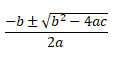The quadratic formula. It can be both impressive and intimidating when students first come across it, this strange mix of algebra, square roots and, most bizarrely, the introduction to many of the ± symbol.

Quadratic equations have been being solved since the times of the Babylonians and Chinese before the birth of Christ; however, these solutions were constructed mostly geometrically. The Indian mathematician Brahmagupta was the first to describe a general formula – though he used words rather than symbols in the AD 600s. It was renowned philosopher and mathematician René Descartes (“I think therefore I am”) who first published the equation in the form we know today in his work La Géométrie.

Though its applications across other areas of science and mathematics are perhaps where it is most useful, the quadratic formula can still be found in everyday life. For example – in simply throwing a ball!

Modelling the path of a ball when it’s thrown is a problem of mechanics; however, solving that problem becomes much simpler when the quadratic formula is used. If you throw a ball directly upwards from a height of 5m with an initial speed of 20m/s, how long will it take to reach the ground? The equations of motion

s = ut + ½at2

Gives us a formula for the relationship between displacement s, initial speed u, acceleration a and time t. When the ball hits the ground, it has been displaced 5m in the opposite direction to its initial movement and so s =-5. Acceleration is due to gravity and is therefore equal to the gravitational constant g ≈ 10. So now the equation has become to solveWe can now use the quadratic formula to solve as a = 5, b = -20 and c = -5 and soAnd so thanks to the quadratic formula, we know it will be just over 4 seconds before the ball hits the ground. This information may seem trivial, but if you’re considering a skydiver instead… suddenly the calculation becomes pretty crucial!